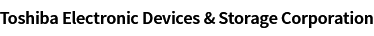### Cross Reference Search

About information presented in this cross reference

The information presented in this cross reference is based on TOSHIBA's selection criteria and should be treated as a suggestion only. Please carefully review the latest versions of all relevant information on the TOSHIBA products, including without limitation data sheets and validate all operating parameters of the TOSHIBA products to ensure that the suggested TOSHIBA products are truly compatible with your design and application.
Please note that this cross reference is based on TOSHIBA's estimate of compatibility with other manufacturers' products, based on other manufacturers' published data, at the time the data was collected.
TOSHIBA is not responsible for any incorrect or incomplete information. Information is subject to change at any time without notice.

### Stock Check & Purchase

Select Product Categories

Select Application

Find everything you need for your next product design. Simply select an application and click through to the block diagram to discover our semiconductor solutions.

New Products / News

Design & Development

Knowledge

# What is the virtual short-circuit (virtual ground) of an op-amp?

A virtual short-circuit (or simply virtual short) refers to a condition of a differential input amplifier such as an op-amp in which its noninverting and inverting inputs have almost the same voltage. This condition is called a virtual short-circuit because the differential inputs have the same voltage even though they are not connected together. This condition is met when a negative-feedback circuit is formed using a differential amplifier with a high open-loop gain.
When the input terminal on one side is grounded to GND as shown in the figure, it is sometimes called virtual ground.
These concepts are helpful for gain calculation.

You can conceptually understand a virtual short as follows.
Typical differential amplifiers have a finite output voltage. The output voltage of an op-amp is equal to its gain multiplied by a difference in voltage between the two inputs. The output voltage of a high-gain circuit becomes extremely large in the event of there being a large difference between the two input voltages. When the output voltage is finite, there is a very little difference in voltage (virtual short) between the two inputs.

Let’s consider this condition using simple calculations. Figure 1 shows a negative-feedback amplifier (inverting amplifier) using an op-amp.
Suppose that it is the ideal op-amp. Then, the following are true:

1. The open-loop gain (AV) is infinite.
2. The input impedance is infinite.
3. The output impedance is zero.

Because the input impedance is infinite, all of the current flowing through R1 (i1) flows through R2.
i1 ＝ (Vi – V) / R1 = (V - Vo) / R2 (1)
The output voltage of the op-amp is given by the equation:
Vo = AV(V+ - V) (2)
From Equation 1 and Equation 2, V+ is calculated as follows:
V+ ＝ {1 + (R1 + R2) / (AV * R1)} × V- - R2 × Vi / (AV × R1) (3)
Because AV is infinite, we obtain V = V.
Hence, the voltage at the negative inverting input (V) is equal to the voltage at the grounded noninverting input (V+).
This condition of the V input is called a virtual short.

Using this concept of a virtual short, the closed loop gain of this circuit can be calculated easily. We can conclude that no current flows to the V input since the V voltage is equal to GND and the input impedance is infinite. Since i1 = i2,
Vi / R2 = Vo / R1 (4)
Let the closed-loop gain of the inverting amplifier be G (= Vo/Vi). Then, G is calculated as R1/R2.

A new window will open
Site Map

Semiconductor Products

Storage Products

Other Products

Company